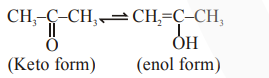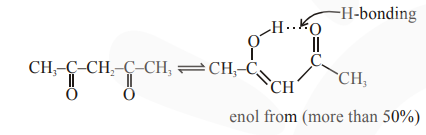# Assertion A : Enol form of acetoneQuestion:

Assertion A : Enol form of acetone $\left[\mathrm{CH}_{3} \mathrm{COCH}_{3}\right]$ exists in $<0.1 \%$ quantity. However, the enol form of acetyl acetone $\left[\mathrm{CH}_{3} \mathrm{COCH}_{2} \mathrm{OCCH}_{3}\right]$ exists in approximately $15 \%$ quantity.

Reason R : enol form of acetyl acetone is stabilized by intramolecular hydrogen bonding, which is not possible in enol form of acetone. Choose the correct statement

1. A is false but R is true

2. Both A and R are true and R is the correct explanation of A

3.  Both A and R are true but R is not the correct explanation of A

4. A is true but R is false

Correct Option: , 2

Solution:enol from of acetone is very less $(<0.1 \%)$